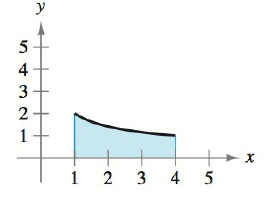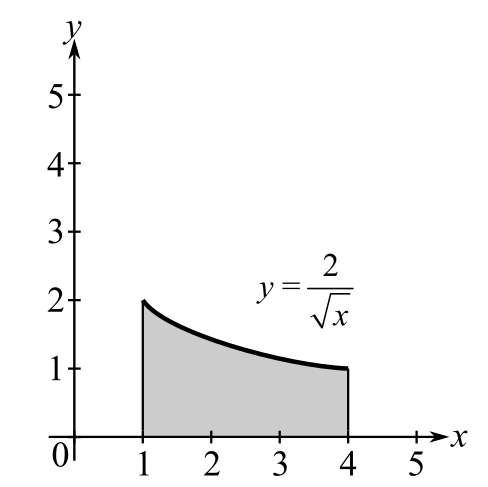Chapter 5.4, Problem 12E### Calculus: An Applied Approach (Min...

10th Edition
Ron Larson
ISBN: 9781305860919

#### Solutions

Chapter
Section### Calculus: An Applied Approach (Min...

10th Edition
Ron Larson
ISBN: 9781305860919
Textbook Problem
1 views

# Finding Area by the Fundamental Theorem In Exercises 9-16, find the area of the region. See Example 2. y = 2 xTo determine

To calculate: The area of the region y=2x in the provided figure.Explanation

Given Information:

The function is y=2x and the provided figure is:

Formula used:

The fundamental theorem of calculus states that,

If f is integrable on interval [a,b] then abf(x)dx=F(b)F(a).

The integration formula is xndx=xn+1n+1+C.

Calculation:

Consider the figure.

To find the area of the region, fundamental theorem of calculus can be used.

Integrate the function from x=1 to x=4 as shown below,

14(2x)dx

This can be written as,

14(2x)dx=14(2x1/2)dx<

### Still sussing out bartleby?

Check out a sample textbook solution.

See a sample solution

#### The Solution to Your Study Problems

Bartleby provides explanations to thousands of textbook problems written by our experts, many with advanced degrees!

Get Started

#### Simplify the expressions in Exercises 97106. (xy)1/3(yx)1/3

Finite Mathematics and Applied Calculus (MindTap Course List)

#### Find the derivative of the function. H(r)=(r21)3(2r+1)5

Single Variable Calculus: Early Transcendentals, Volume I

#### The quadratic approximation for at a = 1 is:

Study Guide for Stewart's Multivariable Calculus, 8th